International
Tables for
Crystallography
Volume D
Physical properties of crystals
Edited by A. Authier

International Tables for Crystallography (2006). Vol. D, ch. 1.1, p. 13

## Section 1.1.4.5.2. Symmetric tensors

A. Authiera*

aInstitut de Minéralogie et de la Physique des Milieux Condensés, Bâtiment 7, 140 rue de Lourmel, 75015 Paris, France
Correspondence e-mail: aauthier@wanadoo.fr

| top | pdf |

#### 1.1.4.5.2.1. Tensors of rank 2

| top | pdf |

A bilinear form is symmetric if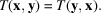Its components satisfy the relations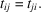The associated matrix, T, is therefore equal to its transpose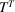: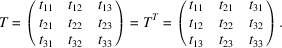In a space with n dimensions, the number of independent components is equal to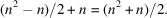#### Examples

 (1) The metric tensor (Section 1.1.2.2) is symmetric because the scalar product is commutative. (2) The tensors representing one of the physical properties associated with the leading diagonal of the matrix of physical properties (Section 1.1.1.4), such as the dielectric constant. Let us take up again the demonstration of this case and consider a capacitor being charged. The variation of the stored energy per unit volume for a variation dD of the displacement is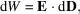where [equation (1.1.3.3)]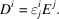Since both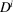and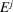are expressed through contravariant components, the expression for the energy should be written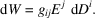If we replaceby its expression, we obtain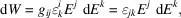where we have introduced the doubly covariant form of the dieletric constant tensor,. Differentiating twice gives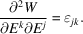If one can assume, as one usually does in physics, that the energy is a `good' function and that the order of the derivatives is of little importance, then one can write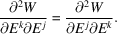As one can exchange the role of the dummy indices, one has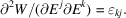Hence one deduces that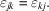The dielectric constant tensor is therefore symmetric. One notes that the symmetry is conveyed on two indices of the same variance. One could show in a similar way that the tensor representing magnetic susceptibility is symmetric. (3) There are other possible causes for the symmetry of a tensor of rank 2. The strain tensor (Section 1.3.1), which is a field tensor, is symmetric because one does not take into account the rotative part of the deformation; the stress tensor, also a field tensor (Section 1.3.1), is symmetric because one neglects body torques (couples per unit volume); the thermal conductivity tensor is symmetric because circulating flows do not produce any detectable effects etc.

#### 1.1.4.5.2.2. Tensors of higher rank

| top | pdf |

A tensor of rank higher than 2 may be symmetric with respect to the indices of one or more couples of indices. For instance, by its very nature, the demonstration given in Section 1.1.1.4shows that the tensors representing principal physical properties are of even rank. If n is the rank of the associated square matrix, the number of independent components is equal to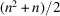. In the case of a tensor of rank 4, such as the tensor of elastic constants relating the strain and stress tensors (Section 1.3.3.2.1), the number of components of the tensor is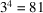. The associated matrix is a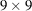one, and the number of independent components is equal to 45.